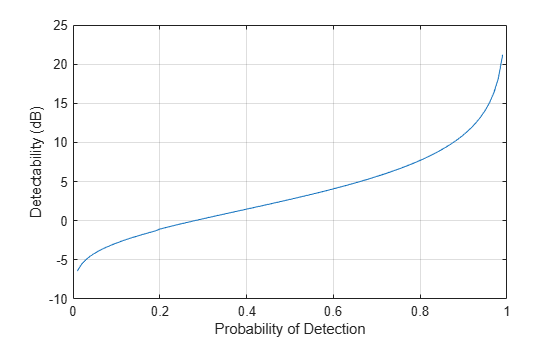# detectability

## Syntax

``D = detectability(PD,PFA)``
``D = detectability(PD,PFA,N)``
``D = detectability(PD,PFA,N,SW)``

## Description

````D = detectability(PD,PFA)` returns the detectability factor of a single radar pulse given the probability of detection `PD` and probability of false alarm `PFA`.The function assumes that you are using a square-law detector and a nonfluctuating target.```
````D = detectability(PD,PFA,N)` returns the detectability factor using the number of pulses for noncoherent integration `N`. .The function assumes that you are using a nonfluctuating target.```

example

````D = detectability(PD,PFA,N,SW)` returns detectability factor using the Swerling case number `SW`. The function assumes you are using a chi-squared distributed target. ```

## Examples

collapse all

Calculate the detectability factor for a Swerling 1 case target. Assume a probability of detection from `0.01–0.99`, probability of false alarm of `1e-6`, and `24` received pulses.

```PFA = 1e-6; PD = 0.01:0.01:0.99; N = 24; D = detectability(PD,PFA,N,'Swerling1'); ```

Plot the detectability factor.

```plot(PD,D) xlabel('Probability of Detection'); ylabel('Detectability (dB)'); grid on```## Input Arguments

collapse all

Probability of detection, specified as a positive scalar in the range `(0,1)` or as a length-J vector with each element in the range `(0,1)`.

Probability of false alarm, specified as a positive scalar in the range `(0,1)` or as a length-K vector with each element in the range `(0,1)`.

Number of pulses for noncoherent integration, specified as a positive scalar.

Swerling case number, specified as one of these

• `'Swerling0'`

• `'Swerling1'`

• `'Swerling2'`

• `'Swerling3'`

• `'Swerling4'`

• `'Swerling5'`

## Output Arguments

collapse all

Detectability factor, returned as a J-by-K matrix in dB with rows corresponding to the number of elements in `PD` and columns corresponding to the number of elements in `PFA`.

## Algorithms

collapse all

### Computation methods used in `detectability` function

The function computes detectability using the computation methods summarized in this table.

Swerling Case Number`PD` is in the range `[0.2, 1-1e-6]` and `PFA` < `1e-4``PD` outside the range `[0.2, 1-1e-6]` or `PFA``1e-4`
0 or 5Shnidman's approximationExact computation
1, 2, 3, 4Barton's universal equation Exact computation

For `Swerling1` and `N` = `1` and `Swerling2` and `N` set to any positive scalar, the function computes the radar detectability factor with no approximation errors using Barton's universal equation. For other Swerling cases, there are small approximation errors when `PD` is in the range `[0.2, 1-1e-6]` and `PFA` < `1e-4`.

## Extended Capabilities

### C/C++ Code GenerationGenerate C and C++ code using MATLAB® Coder™.